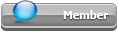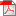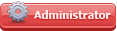## Featured Articles

Check out the latest featured articles.## File Library3# Flow At Tank Discharge - Orifice Vs. Pipe

tank draining pressure drop

3 replies to this topic
|

### #1Steve Hall

Steve Hall

Gold Member

•• ChE Plus Subscriber
•• 167 posts

Posted 23 April 2021 - 07:12 AMFlow from tank formulas.pdf   184.9KB   197 downloadsConsider a storage tank with open discharge.

Most tank draining calculations use an orifice formula with Cd from 0.6 to 0.98 depending on whether the hole is considered to be a sharp-edged orifice up to a stubby pipe (e.g., tank nozzle).

But if the discharge is through a lengthy pipe or through a valve I would think you would use the Darcy equation for pressure drop due to friction with the driving pressure drop being the difference in head from the top of the liquid in the tank to the point where the pipe discharges.

Here's my quandry. I get a much higher flow result using the Darcy equation rather than the orifice equation. I think the Equivalent Length in the Darcy equation needs to incorporate the entrace effect into the pipe such that if the pipe length is zero there is sufficient equivalent length so the result will equal the orifice calculation (same diameters of course). But the K value for entrance pipes is nowhere near high enough to accomplish this.

I must be missing some fundamental here, or my equations have an error, but the same problem exists if I assume a valve is connected to the tank outlet and I simply use the valve flow coefficient to estimate the flow rate.

Any ideas why I have this discrepancy? I've attached my formulas.

Edited by Steve Hall, 23 April 2021 - 07:14 AM.

### #2Bobby Strain

Bobby Strain

Gold Member

•• Members
•• 3,042 posts

Posted 23 April 2021 - 09:20 AM

Welcome back Steve! You will likely get a response from Harvey.

Bobby

### #3breizh

breizh

Gold Member

••• 5,140 posts

Posted 23 April 2021 - 11:10 PM

Hi Steve,

Relation between K and Cv for the application of D.W equation

K*Cv^2=894*d^4  , d internal diameter in inches

Breizh

### #4Steve Hall

Steve Hall

Gold Member

•• ChE Plus Subscriber
•• 167 posts

Posted 29 April 2021 - 06:25 AM

Many thanks to Breizh for pointing me to the right path.

I've determined that if loses for entry and exit to a discharge pipe are included (total K = 1.5), the answer using the equations I posted agree with the orifice model with a Cd of 0.8. In summary, if the tank discharge is consdered through a nozzle on the tank with a project of 150mm or so, the answer using the two approaches (pipe flow or orifice) agree sufficiently for my satisfaction.